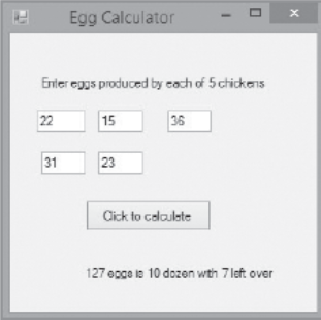Q:

# write a gui program named EggsInteractiveGUI that allows a user to input the number of eggs produced in a month by each of five chicken

write a gui program named EggsInteractiveGUI that allows a user to input the number of eggs produced in a month by each of five chicken. Sum the eggs then display the total in dozens and eggs. for example a total of 127 eggs is 10 dozens of 7 eggs. figure 3-20 shows a typical execution.form1.cs:

``````using System;
using System.Collections.Generic;
using System.ComponentModel;
using System.Data;
using System.Drawing;
using System.Linq;
using System.Text;
using System.Windows.Forms;

namespace WindowsFormsApplication2
{
public partial class Form1 : Form
{
public Form1()
{
InitializeComponent();
}

private void button1_Click(object sender, EventArgs e)
{
int egg1, egg2, egg3, egg4, egg5, total;
egg1 = Int32.Parse(T1.Text.ToString());
egg2 = Int32.Parse(T2.Text.ToString());
egg3 = Int32.Parse(T3.Text.ToString());
egg4 = Int32.Parse(T4.Text.ToString());
egg5 = Int32.Parse(T5.Text.ToString());
total = egg1 + egg2 + egg3 + egg4+egg5;
result.Text = "Total number of eggs produced" + " is " + total / 12 + " dozens and " + total % 12 + " eggs";
}
}
}
``````